### Practice Set 3.4 Arithmetic Progression Class 10th Mathematics Part 1 MHB Solution

Practice Set 3.4
1. On 1st Jan 2016, Sanika decides to save ₹ 10, ₹ 11 on second day, ₹ 12 on third day. If…
2. A man borrows ₹ 8000 and agrees to repay with a total interest of ₹ 1360 in 12 monthly…
3. Sachin invested ina national saving certificate scheme. In the first year he invested ₹…
4. There is an auditorium with 27 rows of seats. There are 20 seats in the first row, 22…
5. Kargil’s temperature was recorded in a week from Monday to Saturday. All readings were…
6. On the world environment day tree plantation programme was arranged on a land which is…
###### Practice Set 3.4
Question 1.

On 1st Jan 2016, Sanika decides to save ₹ 10, ₹ 11 on second day, ₹ 12 on third day. If she decides to save like this, then on 31st Dec 2016 what would be her total saving?

By given information we can form an A.P.

10, 11, 12, 13, ……

Hence, the first term a = 10

Second term t1 = 11

Third term t2 = 12

Thus, common difference d = t2 – t1 = 12 – 11 = 1

Here, number of terms from 1st Jan 2016 to 31st Dec 2016 is,

n = 366

We need to find S366

Now, By using sum of nth term of an A.P. we will find it’s sum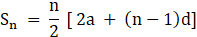Where, n = no. of terms

a = first term

d = common difference

Sn = sum of n terms

Thus, on substituting the given value in formula we get,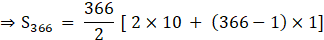⇒S366 = 183 [ 20 + 365]

⇒S366 = 183 × 385

⇒S366 = Rs 70,455

Question 2.

A man borrows ₹ 8000 and agrees to repay with a total interest of ₹ 1360 in 12 monthly instalments. Each instalment being less than the preceding one by ₹ 40. Find the amount of the first and last instalment.

Given: A man borrows = Rs. 8000

Repay with total interest = Rs 1360

In 12 months, thus n = 12

Thus, S12 = 8000 + 1360 = 9360

Each installment being less than preceding one

Thus, d = – 40

We need to find “a”

Now, By using sum of nth term of an A.P. we will find it’s sumWhere, n = no. of terms

a = first term

d = common difference

Sn = sum of n terms

Thus, on substituting the given value in formula we get,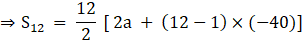⇒ 9360 = 6 [ 2a – 11 × 40]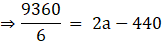⇒ 1560 = 2a – 440

⇒ 1560 + 440 = 2a

⇒ 2a = 2000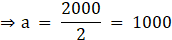Thus, first installment a = Rs. 1000

Now, By using sum of nth term of an A.P. we will find it’s sum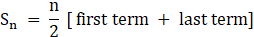Where, n = no. of terms

Sn = sum of n terms

Thus, on substituting the given value in formula we get,

Let a = first term, tn = last term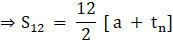⇒ 9360 = 6 [ 1000 + tn]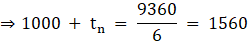⇒ tn = 1560 – 1000 = 560

Thus, last installment tn = 560

Question 3.

Sachin invested ina national saving certificate scheme. In the first year he invested ₹ 5000, in the second year ₹ 7000, in the third year ₹ 9000 and so on. Find the total amount that he invested in 12 years.

By given information we can form an A.P.

5000, 7000, 9000, ……

Hence, the first term a = 5000

Second term t1 = 7000

Third term t2 = 9000

Thus, common difference d = t2 – t1 = 9000 – 7000 = 2000

Here, number of terms n = 12

We need to find S12

Now, By using sum of nth term of an A.P. we will find it’s sumWhere, n = no. of terms

a = first term

d = common difference

Sn = sum of n terms

Thus, on substituting the given value in formula we get,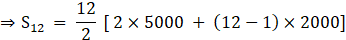⇒S12 = 6 × [ 10,000 + 11 × 2000]

⇒S12 = 6 × [10,000 + 22,000]

⇒S12 = 6 × 32,000

⇒S12 = Rs. 192000

Question 4.

There is an auditorium with 27 rows of seats. There are 20 seats in the first row, 22 seats in the second row, 24 seats in the third row and so on. Find the number of seats in the 15th row and also find how many total seats are there in the auditorium?

Given: first term a = 20

Second term t1 = 22

Third term t2 = 24

Common difference d = t2 – t1 = 24 – 22 = 2

We need to find t15 thus n = 15

Now, By using nth term of an A.P. formula

tn = a + (n – 1)d

where n = no. of terms

a = first term

d = common difference

tn = nth terms

On substituting all value in nth term of an A.P.

⇒ t15 = 20 + (15 – 1) × 2

⇒ t15 = 20 + 14 × 2

⇒ t15 = 20 + 28 = 48

We have been given that, there are 27 rows in an auditorium

Thus, we need to find total seats in auditorium i.e. S27

Now, By using sum of nth term of an A.P. we will find it’s sumWhere, n = no. of terms

a = first term

d = common difference

Sn = sum of n terms

Thus, on substituting the given value in formula we get,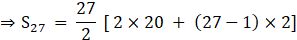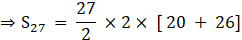⇒S27 = 27 × 46

⇒S27 = 1242

Question 5.

Kargil’s temperature was recorded in a week from Monday to Saturday. All readings were in A.P. The sum of temperatures of Monday and Saturday was 5° C more than sum of temperatures of Tuesday and Saturday. If temperature of Wednesday was – 30° celsius then find the temperature on the other five days.

Let Monday be the first term i.e. a = t1

Let Tuesday be the second term i.e t2

Let Wednesday be the third term i.e t3

Let Thursday be the fourth term i.e t4

Let Friday be the fifth term i.e t5

Let Saturday be the sixth term i.e t6

Given: t1 + t6 = 5 + (t2 + t6)

⇒ a = 5 + (t2 + t6) – t6

⇒ a = 5 + t2 …..(1)

We know that,

Now, By using nth term of an A.P. formula

tn = a + (n – 1)d

where n = no. of terms

a = first term

d = common difference

tn = nth terms

Thus, t2 = a + (2 – 1) d

⇒ t2 = a + d

Now substitute value of t2 in (1) we get,

⇒ a = 5 + (a + d)

⇒ d = a – 5 – a = – 5

Given: t3 = – 30°

Thus, t3 = a + (3 – 1) × ( – 5)

⇒ – 30 = a + 2 × ( – 5)

⇒ – 30 = a – 10

⇒ a = – 30 + 10 = – 20°

Thus, Monday, a = t1 = – 20°

Using formula tn + 1 = tn + d

We can find the value of the other terms

Tuesday, t2 = t1 + d = – 20 – 5 = – 25°

Wednesday, t3 = t2 + d = – 25 – 5 = – 30°

Thursday, t4 = t3 + d = – 30 – 5 = – 35°

Friday, t5 = t4 + d = – 35 – 5 = 40°

Saturday, t6 = t5 + d = – 40 – 5 = – 45°

Thus, we obtain an A.P.

– 20°, – 25°, – 30°, – 35°, – 40°, – 45°

Question 6.

On the world environment day tree plantation programme was arranged on a land which is triangular in shape. Trees are planted such that in the first row there is one tree, in the second row there are two trees, in the third row three trees and so on. Find the total number of trees in the 25 rows.

First term a = 1

Second term t1 = 2

Third term t3 = 3

Common difference d = t3 – t2 = 3 – 2 = 1

We need to find total number of trees when n = 25

Thus, By using sum of nth term of an A.P. we will find it’s sumWhere, n = no. of terms

a = first term

d = common difference

Sn = sum of n terms

We need to find S25

Thus, on substituting the given value in formula we get,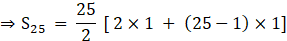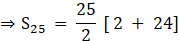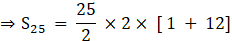⇒S25 = 25 × 13 = 325

## PDF FILE TO YOUR EMAIL IMMEDIATELY PURCHASE NOTES & PAPER SOLUTION. @ Rs. 50/- each (GST extra)

SUBJECTS

HINDI ENTIRE PAPER SOLUTION

MARATHI PAPER SOLUTION
SSC MATHS I PAPER SOLUTION
SSC MATHS II PAPER SOLUTION
SSC SCIENCE I PAPER SOLUTION
SSC SCIENCE II PAPER SOLUTION
SSC ENGLISH PAPER SOLUTION
SSC & HSC ENGLISH WRITING SKILL
HSC ACCOUNTS NOTES
HSC OCM NOTES
HSC ECONOMICS NOTES
HSC SECRETARIAL PRACTICE NOTES

2019 Board Paper Solution

HSC ENGLISH SET A 2019 21st February, 2019

HSC ENGLISH SET B 2019 21st February, 2019

HSC ENGLISH SET C 2019 21st February, 2019

HSC ENGLISH SET D 2019 21st February, 2019

SECRETARIAL PRACTICE (S.P) 2019 25th February, 2019

HSC XII PHYSICS 2019 25th February, 2019

CHEMISTRY XII HSC SOLUTION 27th, February, 2019

OCM PAPER SOLUTION 2019 27th, February, 2019

HSC MATHS PAPER SOLUTION COMMERCE, 2nd March, 2019

HSC MATHS PAPER SOLUTION SCIENCE 2nd, March, 2019

SSC ENGLISH STD 10 5TH MARCH, 2019.

HSC XII ACCOUNTS 2019 6th March, 2019

HSC XII BIOLOGY 2019 6TH March, 2019

HSC XII ECONOMICS 9Th March 2019

SSC Maths I March 2019 Solution 10th Standard11th, March, 2019

SSC MATHS II MARCH 2019 SOLUTION 10TH STD.13th March, 2019

SSC SCIENCE I MARCH 2019 SOLUTION 10TH STD. 15th March, 2019.

SSC SCIENCE II MARCH 2019 SOLUTION 10TH STD. 18th March, 2019.

SSC SOCIAL SCIENCE I MARCH 2019 SOLUTION20th March, 2019

SSC SOCIAL SCIENCE II MARCH 2019 SOLUTION, 22nd March, 2019

XII CBSE - BOARD - MARCH - 2019 ENGLISH - QP + SOLUTIONS, 2nd March, 2019

HSC Maharashtra Board Papers 2020

(Std 12th English Medium)

HSC ECONOMICS MARCH 2020

HSC OCM MARCH 2020

HSC ACCOUNTS MARCH 2020

HSC S.P. MARCH 2020

HSC ENGLISH MARCH 2020

HSC HINDI MARCH 2020

HSC MARATHI MARCH 2020

HSC MATHS MARCH 2020

SSC Maharashtra Board Papers 2020

(Std 10th English Medium)

English MARCH 2020

HindI MARCH 2020

Hindi (Composite) MARCH 2020

Marathi MARCH 2020

Mathematics (Paper 1) MARCH 2020

Mathematics (Paper 2) MARCH 2020

Sanskrit MARCH 2020

Important-formula

THANKS